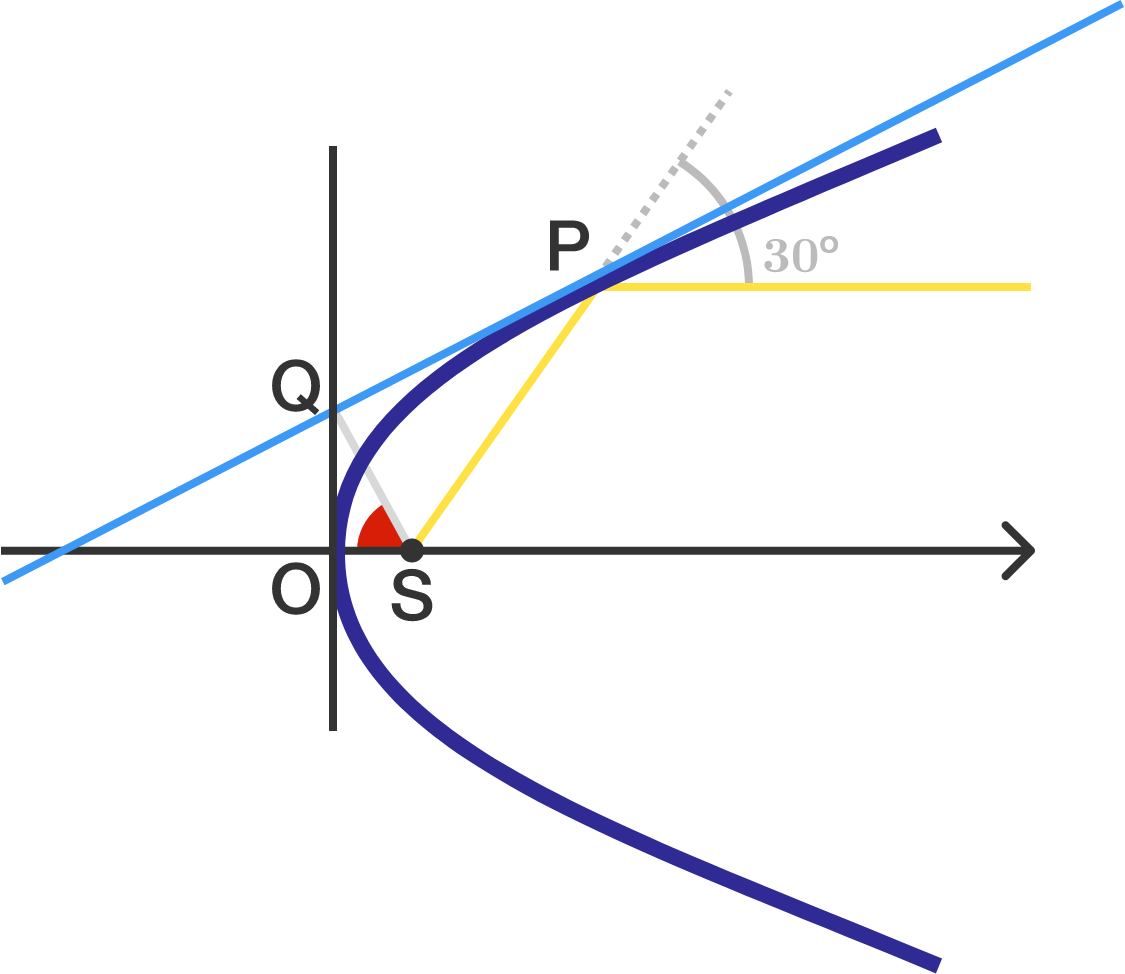# Geometry in Parabola!

Geometry Level 2

Consider a parabolic mirror with vertex $O$. A light ray originating from focus $S$ of the parabola, strikes the mirror at a point $P$ such that it gets deviated by $30^{\circ}$ after reflection.

Now, if we draw a tangent at point $P$ to the same parabola, it intersects the tangent at vertex at point $Q$. Find $\angle OSQ$ (in degrees).The image is not drawn to scale.

×

Problem Loading...

Note Loading...

Set Loading...